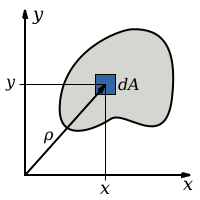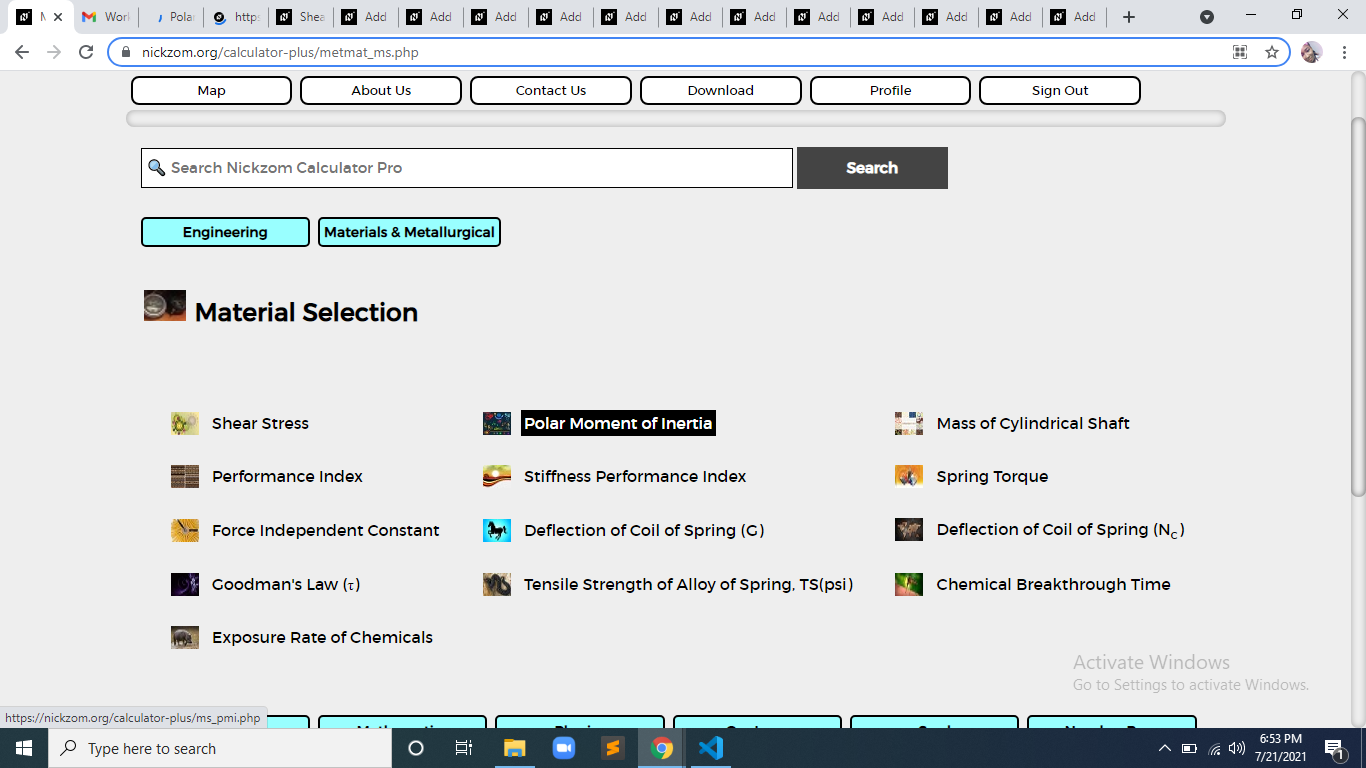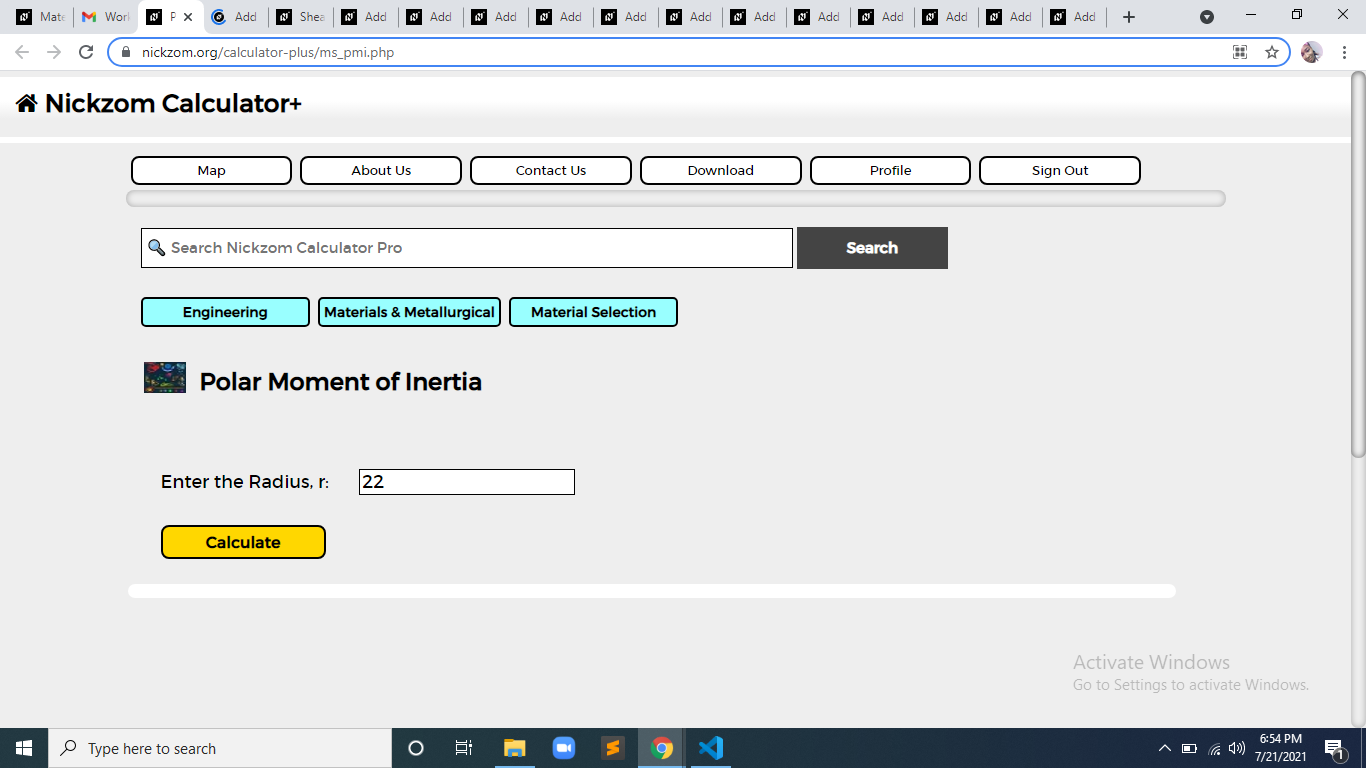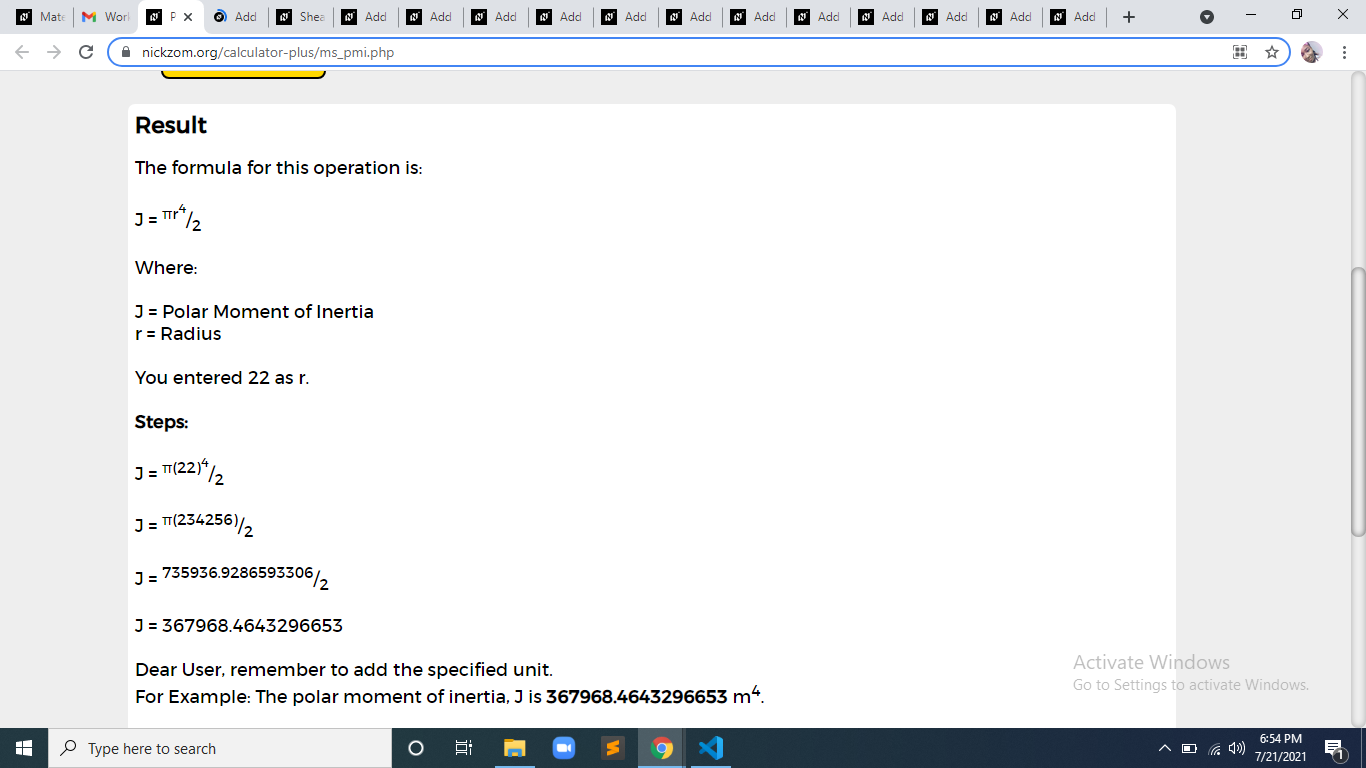# How to Calculate and Solve for Polar Moment of Inertia | Material SelectionThe image above represents polar moment of inertia.

To compute for polar moment of inertia, one essential parameter is needed and this parameter is Radius (r).

The formula for calculating polar moment of inertia:

J = πr4/2

Where:

J = Polar Moment of Inertia

Let’s solve an example;
Find the polar moment of inertia when the radius is 22.

This implies that;

J = πr4/2
J = π(22)4/2
J = π(234256)/2
J = 735936.928/2
J = 367968.46

Therefore, the polar moment of inertia is 367968.46 m4.

Calculating the Radius when the Polar Moment of Inertia is Given.

r = 4√(2J / π)

Where;

J = Polar Moment of Inertia

Let’s solve an example;
Given that the polar moment of inertia is 30. Find the radius?

This implies that;

J = Polar Moment of Inertia = 30

r = 4√(2J / π)
r = 4√(2 x 30 / π)
r = 4√(60 / π)
r = 4√19.09
r = 17.47

Therefore, the radius is 17.47 m.

Nickzom Calculator – The Calculator Encyclopedia is capable of calculating the polar moment of inertia.

To get the answer and workings of the polar moment of inertia using the Nickzom Calculator – The Calculator Encyclopedia. First, you need to obtain the app.

You can get this app via any of these means:

To get access to the professional version via web, you need to register and subscribe for NGN 2,000 per annum to have utter access to all functionalities.
You can also try the demo version via https://www.nickzom.org/calculator

Apple (Paid) – https://itunes.apple.com/us/app/nickzom-calculator/id1331162702?mt=8
Once, you have obtained the calculator encyclopedia app, proceed to the Calculator Map, then click on Materials and Metallurgical under Engineering.Now, Click on Material Selection under Materials and MetallurgicalNow, Click on Polar Moment of Inertia under Material SelectionThe screenshot below displays the page or activity to enter your valus, to get the answer for the polar moment of inertia according to the respective parameter which is the Radius (r).Now, enter the value appropriately and accordingly for the parameter as required by the Radius (r) is 22.Finally, Click on CalculateAs you can see from the screenshot above, Nickzom Calculator– The Calculator Encyclopedia solves for the polar moment of inertia and presents the formula, workings and steps too.